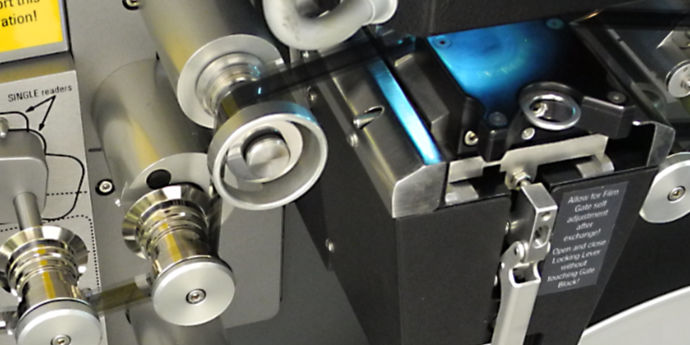# A Film Restoration Diary## Home-Grown Jitter Stabilisation — Part 3 — Image Correlation to Detect Movements

In the last couple of posts dealing with “Home-Grown Jitter Stabilisation”, we established:

• The jitter comprises displacement, rotation and vertical scaling.
• There is a white dot (partially) visible in each of the four corners of each scanned film frame. These dots move together with the video raster.
• If we can measure the frame-to-frame movement of these dots, we can calculate and then correct (i.e. remove) the jitter.

So now we come to the topic of how to measure the frame-to-frame movement of these white dots.

This is how we will do it:

• Adopt a particular frame as a reference frame against which other frames will be compared.
• Sample (i.e. make copies of) small areas containing the white dots in each of the four corners of each frame.
• Compare these small “white dot” images with the same images sampled from the reference frame.
• This can tell us the amount of movement of each corner white dot relative to the reference frame.

But how to compare these small sample images and measure the relative movement of the white dots? To do this we need to use image correlation.

Think about it this way: Imagine you have two pieces of black paper of the same size. Each piece of paper has a small circular hole. The holes are the same size, but in different locations on each piece of paper. The challenge is to work out the relative location of the two holes.

We can do this by placing the pieces of paper on a light table and systematically moving the top piece of paper relative to the bottom piece. At some point the two holes will line up. When the holes are perfectly aligned, the amount of light coming through the holes will be at a maximum. But if the holes are only partially aligned, then the amount of light will be less. When there is no overlap of the holes at all, there will be no light transmitted.

By finding the place where the maximum amount of light is transmitted, we can measure the relative positions of the two pieces of paper and hence calculate the relative positions of the holes in each piece of paper.

This simple example more or less explains how image correlation works, but instead of dealing with holes in pieces of paper, we are dealing with digital images and mathematical computation is used to determine the location when the alignment of the two images (i.e. in our case two white dots) is optimum. At this point what is called the correlation of the two images — equivalent to the amount of light transmitted in our paper example — is at a maximum.

This process of correlation can yield a horizontal (x) and vertical (y) offset measurement in pixels corresponding to the offset of the white dots.

Problem solved.

In the next post we will look at how the measured offsets of each corner dot can be used to calculate the displacement, rotation and vertical stretch of the test frame relative to the reference frame.